﻿ Impact of V2G on Distribution Feeder: An Energy Loss Reduction Approach

### Impact of V2G on Distribution Feeder: An Energy Loss Reduction Approach

Uwakwe C. Chukwu, Satish M. MahajanOPEN ACCESSPEER-REVIEWED

## Impact of V2G on Distribution Feeder: An Energy Loss Reduction Approach

Uwakwe C. Chukwu1,, Satish M. Mahajan2

1Department of Industrial & Electrical Engineering Technology of South Carolina State University, U.S.A.

2Electrical and Computer engineering, Tennessee Technological University, Cookeville, TN, U.S.A.

### Abstract

As Vehicle-to-Grid (V2G) concept convenes strong interests among automakers, electric utility owners, and policy makers, it is critical to investigate the potential impacts of V2G on the electric distribution system. Such study, together with other benefits of V2G, may encourage utility support for V2G commercialization. This paper focuses on a pressing technical issue associated with the penetration of V2G into the electric distribution network- the energy loss in the feeders. Mathematical models are formulated to investigate how V2G reactive power injection and different load patterns can impact energy loss on a distribution system. It is shown that loss reduction can be greatly influenced by reactive loading factor, the pattern of loading, the amount of V2G reactive power injection, and the position of V2G parking lot on the feeder segment. Useful results are obtained, with a promise that more than 95% energy loss reduction (relative to energy loss in the system without V2G installed) is possible by optimally locating a V2G parking lot along the feeder. The results in this study may serve as a nomogram to determine energy loss at the planning stage of installing a V2G parking lot on a distribution feeder segment.

### At a glance: Figures

• Chukwu, Uwakwe C., and Satish M. Mahajan. "Impact of V2G on Distribution Feeder: An Energy Loss Reduction Approach." International Transaction of Electrical and Computer Engineers System 2.1 (2014): 19-27.
• Chukwu, U. C. , & Mahajan, S. M. (2014). Impact of V2G on Distribution Feeder: An Energy Loss Reduction Approach. International Transaction of Electrical and Computer Engineers System, 2(1), 19-27.
• Chukwu, Uwakwe C., and Satish M. Mahajan. "Impact of V2G on Distribution Feeder: An Energy Loss Reduction Approach." International Transaction of Electrical and Computer Engineers System 2, no. 1 (2014): 19-27.

 Import into BibTeX Import into EndNote Import into RefMan Import into RefWorks

### 1. Introduction

Conventional plug-in-electric vehicle (PHEV) may commute on electric mode by utilizing energy from the on-board battery, which is often charged. A PHEV with the capability for both charging of battery and discharging to the electric grid when needed, otherwise called Vehicle-to-Grid (V2G), is receiving considerable attention within the research and industrial community.V2G application is transformational and revolutionary in that it precipitates the confluence of transportation and electric power system, thereby introducing electricity as a meaningful automotive fuel. This concept requires charging and discharging of V2G batteries. Unfortunately, uncoordinated and random V2G charging can cause increased energy losses, overloads and voltage fluctuations . The delivery of electrical power from sources to the load centers is always accompanied with energy losses in the feeder lines. With the rising research activities justifying the penetration of V2G into the distribution system, an extremely important technical issue emerges: What impact does penetration of V2G in the distribution network have on the energy loss. The problem of energy loss in distribution system has attracted the attention of system operators for several decades [1-6]. Some studies have determined that the total T & D losses in an ideal power system are approximately 3 to 6% of the total generated energy due to Joule effect . About 80-90% of the total T & D losses take place in the distribution system . The total T & D losses in the year 2005 amounted to 239 million MWh, or 6.1% of the net generation in the US . Energy loss management is a very important sequel to its direct relationship with economic operations and the overall efficiency of the distribution utilities. The economic and technical aspects of using V2G to support the electric grid have been discussed in [7, 8, 9, 10, 11].

V2G facilities can be used for ancillary services, like spinning reserve, peak load and reactive power compensations . However, spinning reserve and peak load operations require injection of real power to the grid, which have been known to degrade battery state of charge and life expectancy. It has been investigated that using V2G battery to supply reactive power compensation does not cause any degradation at all on the battery life, since the dc link capacitor is sufficient to supply full reactive power . Hence, in this paper, active power injection is not considered based on the technical issues associated with active power injection using V2G battery (this is a major concern among V2G owners [8, 11]). Analysis of the possible size of the V2G parking lot (in terms of power injection) was made. Reactive power compensation ratio was used to represent the different parking lot reactive power capacities.

This paper is organized as follows: - In section II, the modeling of energy loss reduction in distribution system is presented. Section III presents the numerical results and analysis using different operational scenarios. Conclusions are made in section IV.

### 2. Modeling of Power Loss Reduction

This paper investigates the impact of V2G parking lots on the energy loss reduction, taking into consideration various scenarios of loading pattern, capacity injection, V2G location relative to the feeder source, and load factor.

The per unit energy loss reduction ΔELS_V2G in a three phase distribution line segment is defined as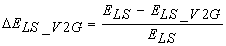Where ELS and ELS_V2G are respectively the energy losses before V2G was installed, and after V2G was installed. The per unit energy loss reduction for n number of V2G parking lots in a feeder line may be quantified as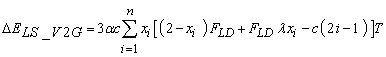(1)

where FLD is the reactive load factor (FLD = Q / S), and T is the normalized total period of time that the V2G parking lot is supplying reactive power. The quantity, xi, represents the position of the ith V2G parking lot, while the load pattern, λ, defines the loading characteristic of the line segment (λ = 0 represents uniformly distributed load and while λ = 1 represents lumped loads). The variation of the load is represented by ‘λ’. A mix of uniformly distributed load and lumped loads can be covered in the range 0 < λ < 1 .

From (1), the following parameters are defined ,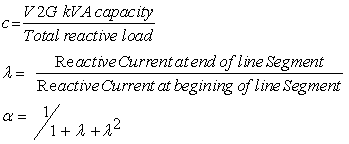For a single V2G parking lot structure installed along the feeder line (such as presented in Figure 1), the per unit energy loss reduction can be established from (1) as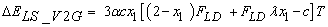(2)

The optimal location of V2G for maximum energy loss reduction may be computed by taking the derivative of the above equation with respect to x1 and equating to zero. Thus, taking derivative of (2),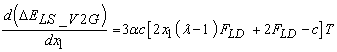(3)

Equating the above equation to zero and solving for x1 results in the optimal V2G location, x1,opt, as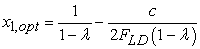(4)

Substituting (4) into (2) results in an optimal per unit energy loss reduction,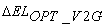, for a V2G, as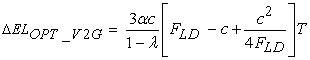(5)

Using (5) and fixing any four of the five parameters (i.e., x1, c, λ, FLD and T), it is possible to investigate energy loss reduction as a function of the fifth parameter. It is based on this consideration that a nomogram was developed with the aim to guide distribution system planners to know a priori the impact that a single V2G parking lot (located on a line segment) will have on energy loss, while actively considering the five parameters. The computer programs for this purpose were written in MATLAB, and simulation results are presented in the next section.

### 3. Numerical Results

The results of the per unit energy loss reduction due to a single V2G parking lot structure on distribution feeder line segment are presented in this section. In order to make a comprehensive study of the impact of V2G on energy loss, various parameter categorizations are methodically followed as presented in Table 1. In the table, ‘contour’ simply refers to a plot (in the simulation Figures). In all the simulation results, each contour is distinguished by a parameter value (value appearing on contours). Also, the column indicated as ‘title’ in the table indicates two global fixed parameters. For any subplot, there are 2 global parameters; however, the characteristics in a subplot are distinguished from another by a changing global parameter. For example, in Category 1, the effects of energy loss reduction as V2G location varies for various reactive compensation ratios and load patterns are presented in Figure 2 through Figure 7. In this case (Category 1), reactive compensation ratio is the contour, while as λ and FLD are the global fixed parameters (the title). The analysis of energy loss reduction is presented according to category classification, starting from Category 1. The vertical axis of the plots refers to per unit energy loss reduction (not energy loss)-this has been defined in section II. For example, energy loss reduction of 0.2 p. u means that V2G injecting reactive power will result in 20% less energy loss than same network without V2G.

#### Table 1. Parameter Categorizations for Result SimulationsDownload asPowerPoint Slide

Veiw figureView current table in a new window

3.1. Category 1

The results in Figure 2 show that energy loss reduction increases with increasing location of V2G parking lot (away from the beginning of the line segment), although a limiting loss reduction is attained as x1 increases. From mathematical point of view, substituting appropriate parameters into (4) indicates the optimal location at which limiting energy loss defined in (5) will take place. For example, in sub-plot (1, 1) of Figure 2 where λ = 0 and FLD = 0.2, substituting λ = 0 and FLD = 0.2 into (4) and assuming c = 0.2 (a contour) results in 0.5 p.u optimal placement of the V2G on the feeder line. However, substituting these parameters in (5) results in optimal energy loss reduction of 0.03. From power system perspective, it means that a system planner or operator desiring reactive compensation ratio of 0.2, a uniformly distributed load operation (λ = 0) and reactive load factor (FLD) of 0.2 must install the V2G parking lot at 0.5 p.u in order to achieve a maximum loss reduction of 3%. Should the V2G parking lot be located anywhere else on the feeder, the loss reduction will be less than 3%. Hence, system planners or operators can only have one V2G location for any given operational choice (λ, c, and FLD) in order to achieve maximum energy loss reduction. The developed nomograms can be used to read-off the magnitudes of the energy loss for different operation scenarios (represented as ‘category’ in this study). In a real-life situation, it may be difficult (or even impossible), due to land terrain and existing permanent infrastructures, to locate a V2G parking lot at the optimum location. This situation will definitely lead to sub-optimum location and less-than-maximum energy loss reduction.

Figure 2. Energy Loss reduction with any V2G Location for various reactive compensation ratio and load patterns (taking FLD = 0.2)

It is further observed in that energy loss reduction increases as λ → 1 (i.e., as load pattern becomes lumped). Finally, the results reveal that as λ → 1, the characteristics tend to linearity. In Figure 2, FLD is assumed to be 0.2; however, the results when FLD is taken to be 0.2. 0.4, 0.6, 0.8 and 1.0 are similarly presented in Figure 3 through Figure 6. It can be concluded from Figure 3 through Figure 6 that increasing FLD leads to increasing energy loss reduction. However, operating at high FLD will compromise the voltage stability of the system. In Figure 2 and Figure 3, it is seen that there are limited range of reactive compensations to operate at for FLD of 0.2 and 0.4. This implies that it is operationally infeasible to operate the system at high reactive compensations when FLD is considerably low.

Figure 3. Energy Loss reduction with any V2G Location for various reactive compensation ratio and load patterns (taking FLD = 0.4)
Figure 4. Energy Loss reduction with any V2G Location for various reactive compensation ratio and load patterns (taking FLD = 0.6)
Figure 5. Energy Loss reduction with any V2G Location for various reactive compensation ratio and load patterns (taking FLD = 0.8)
Figure 6. Energy Loss reduction with any V2G Location for various reactive compensation ratio and load patterns (taking FLD = 1.0)
3.2. Category 2

The energy loss reduction due to V2G reactive compensation ratio for various V2G Locations and different representative load patterns (taking FLD = 0.2, 0.4, 0.6, 0.8 and 1.0) are presented in Figure 7 to Figure 11.

Figure 7. Energy Loss reduction with any V2G reactive compensation ratio for various V2G Locations and different representative load patterns (FLD = 0.2)
Figure 8. Energy Loss reduction with any V2G reactive compensation ratio for various V2G Locations and different representative load patterns (FLD = 0.4)
Figure 9. Energy Loss reduction with any V2G reactive compensation ratio for various V2G Locations and different representative load patterns (FLD = 0.6)
Figure 10. Energy Loss reduction with any V2G reactive compensation ratio for various V2G Locations and different representative load patterns (FLD = 0.8)

One can observe that at FLD = 0.2, energy loss reduction is a negative quantity, i.e., the feeder loss increases. It is noticed in Figure 7 and Figure 8 that the extent of increase in feeder loss depends on the magnitude of λ, with higher loss as λ → 0. In Figure 9, it is seen that both energy loss reduction and energy loss increase (i.e., negative energy loss reduction, especially in the range λ ≤ 0.4.) are possible. This situation of increase in feeder loss is operationally undesirable, and should be avoided. The results presented in this category show that as FLD increases from 0.2 to 1.0, the energy loss reduction improves, and eventually becomes positive (i.e., the feeder loss decreases). Figure 10 shows that energy loss reduction improved markedly in all operations, except in subplots (1, 1), where the location of the V2G parking lot at x1 = 0.9 and x1 = 1 led to increase in feeder loss when c > 0.8. Finally, in Figure 11, it is observed that the best system operation is represented in all cases considered, since no increase in feeder loss is seen in the results.

Figure 11. Energy Loss reduction with any V2G reactive compensation ratio for various V2G Locations and different representative load patterns (FLD = 1.0)
3.3. Category 3

The simulation results for energy loss reduction with any V2G location for various representative load patterns and reactive compensation ratios are presented in Figure 12 through Figure 16 (for 0 ≤ FLD ≤ 1). It is further observed that at FLD = 0.2, energy loss reduction is a negative quantity.

In Figure 12, it is seen that the feeder loss increases in the feeder as x1 → 1 for any given λ and c. The impact of progressively increasing FLD on energy loss reduction is presented in Figure 13 to Figure 16. It is observed that energy loss reduction progressively increases as FLD is increased. It is also observed that limiting value of energy loss reduction sets in as FLD → 1, and the characteristic becomes more parabolic at higher reactive compensations. In Figure 16, it is observed that considerable energy loss reduction is achieved in all the cases considered. Hence, again, results in Category 3 analysis show that it is recommended to operate at high FLD.

Figure 12. Energy Loss reduction with any V2G location for various representative load patterns and reactive compensation ratio (FLD = 0.2)
Figure 13. Energy Loss reduction with any V2G location for various representative load patterns and reactive compensation ratio (FLD = 0.4)
Figure 14. Energy Loss reduction with any V2G location for various representative load patterns and reactive compensation ratio (FLD = 0.6)
Figure 15. Energy Loss reduction with any V2G location for various representative load patterns and reactive compensation ratio (FLD = 0.8)
Figure 16. Energy Loss reduction with any V2G location for various representative load patterns and reactive compensation ratio (FLD = 1.0)
3.4. Category 4

The results of Category 4 analysis are presented in Figure 17 through Figure 21. It is noticed in the results of this category that energy loss reduction may decrease or increase depending on the value of x1 (contour), FLD and c. As can be seen, most of the cases investigated resulted in negative energy loss reduction (i.e., increase in energy loss). It is also observed that energy loss reduction progressively increases as FLD is increased from 0.2 to 1.0. In Figure 21, it is observed that considerable energy loss reduction is obtained at x1 = 1.0. Analysis shows that it is recommended to operate at high FLD.

Figure 17. Energy Loss reduction with any representative load patterns for various V2G locations and reactive compensation ratio (FLD = 0.2)
Figure 18. Energy Loss reduction with any representative load patterns for various V2G locations and reactive compensation ratio (FLD = 0.4)
Figure 19. Energy Loss reduction with any representative load patterns for various V2G locations and reactive compensation ratio (FLD = 0.6)
Figure 20. Energy Loss reduction with any representative load patterns for various V2G locations and reactive compensation ratio (FLD = 0.8)
Figure 21. Energy Loss reduction with any representative load patterns for various V2G locations and reactive compensation ratio (FLD = 1.0)

In all the analysis presented so far, no consideration was made for optimum energy loss reduction, which is known to result in the most efficient system operation. Optimum energy loss reduction obviously eliminates any operation that will lead to negative energy loss reduction. The optimum V2G reactive compensation ratio required to provide optimum energy loss reduction is given by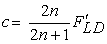(6)

Equation (6) shows that as the number of V2G parking lots approaches infinity, the optimum V2G reactive compensation ratio approaches reactive load factor, FLD. Figure 22 shows the relationship between optimum V2G reactive compensation ratio, c, and reactive load factor, FLD (for n = 1), necessary to obtain optimal energy loss reduction. From (5), the optimum energy loss reduction with any magnitude of reactive injection from a V2G parking lot located at the optimum location for various reactive load factors is calculated and results presented in Figure 22. From the results, it is observed that as λ → 1, the optimum energy loss reduction increases. More so, the energy loss reduction increases as FLD increases. It is evident from this result that for all values of λ, when reactive load factors are 0.2 and 0.45, the use of V2G parking lot injecting reactive power with corrective ratios of 0.4 and 0.9, respectively, results in a zero energy loss reduction. It is critical operational consideration for system planners to know a priori the level of injection that will result in zero energy loss condition.

Figure 22. Optimal Energy Loss with any V2G reactive compensation ratio and various load pattern for a V2G facility at optimum site (FLD = 0.2 to 0.95 pu).

From Figure 6, Figure 11, Figure 16, Figure 21, and Figure 22, it could be observed that a 95% loss reduction is possible. Although it may not be possible in a real word scenario to optimize every parameter of the power system having a V2G in it, attempts to optimize under unavoidable constraints could be directed to operate at lowest possible FLD, and highest possible c, λ and x1. Basically, from the results, increasing FLD, lambda, c and x1 can maximize energy loss reduction (i.e. reduce energy loss). However, a further work may address a limit for increasing FLD, lambda, c and x1 that brings about enhanced energy loss reduction, without compromising voltage profiles.

### 4. Conclusions

This paper investigated the impact of V2G parking lot facility (injecting only reactive power into the distribution system) on energy loss reduction. The model employed for this study quantified the amount of energy loss reduction, considering the following variables: load pattern, the level of V2G reactive injection, the location of V2G parking lot on the feeder as well as the reactive load factor. The results indicated that more than 95% energy loss reduction (relative to power loss in the system without V2G installed) is possible by optimally locating a V2G parking lot along the feeder. Location of V2G facility as well as the capacity injection and reactive load factor have critical impact on energy loss reduction. In real-world situation, it may be difficult-if not impossible-to locate a V2G parking lot at the optimum location. With the developed nomogram, it is possible for distribution system planners to know, before hand, the impacts V2G parking lot will have on the energy loss of a distribution network. The work also has the potential to serve as a tool for identifying operational corrective ratios necessary for achieving zero energy loss in the distribution networks.

### Acknowledgement

The authors gratefully acknowledge the funding support for this research from office of Research and graduate studies, Tennessee Tech. University, Cookeville, TN 38501, U.S.A.

### References

  S. Deilami, A.s. Masoum, P.S. Moses, M.A.S. Masoum, “Real-Time Coordination of Plug-In Electric Vehicle Charging in Smart Grids to Minimize Power Losses and Improve Voltage Profile” IEEE Trans. on Smart Grid, Vol. 2, Iss.3, pp. 456-467, 2011.In article CrossRef  N. Jelani, M. Molinas, “Loss minimization in AC distribution system with high share of power electronic loads providing ancillary reactive power”, IEEE Power Tech, Trondheim , pp. 1-6, 2011.In article  L. G. Meegahapola, S. R. Abbott, D. J. Morrow, T. Littler, D. Flynn, “Optimal allocation of distributed reactive power resources under network constraints for system loss minimization IEEE PES GM. pp. 1-7, 2011.In article  L. Ramesh, S.P. Chowdhury, S. Chowdhury, A.A. Natarajan, C. Gaunt, “Minimization of Power Loss in Distribution Networks by Different Techniques” Int. J. of Electrical &Electronic Eng. vol.3, (9), pp. 511-527, 2009.In article  K. V. S. Ramachandra Murthy and M. Ramalinga Raju, “Electrical Energy Loss in Rural Distribution Feeders-A Case Study” ARPN J. of Eng. and Applied Science, Vol. 4, No. 2, pp. 33-36, 2009.In article  [Online]. Available: http://www04.abb.com/global/seitp/seitp202.nsf.In article  W. Kempton, S. Letendre, “Electric vehicles as a new source for electric utilities,” Transportation. Research Part D, vol. 2, (3), pp. 157-175, 1997.In article CrossRef  W. Kempton and J. Tomic, “Vehicle-to-grid power fundamentals: Calculating Capacity and net revenue,” Journal of Power Sources, vol. 144, no. 1, pp. 268-279, 2005.In article CrossRef  S. Letendre and W. Kempton. 2002. “The V2G Concept: A new Model for Power?” Public Utilities Fortnightly, pp. 17-26, 2002.In article  A.Y. Saber, G.K. Venayagamoorthy, “Optimization of vehicle-to-grid scheduling in constrained parking lots” Power & Energy Society General Meeting, 2009, pp 1-8.In article  J. Tomi´, W. Kempton, “Using fleets of electric-drive vehicles for grid support” Energy Policy, vol. 36, pp. 3578-3587, 2008.In article  T. Gonen, Electric Power Distributed System Engineering, 2nd printing Ed. New York: McGraw-Hill, 1987, pp 414-435.In article  M. C. Kisacikoglu, B. Ozpineci, L. M. Tolbert, “Examination of a PHEV bidirectional charger for V2G reactive power compensation.” in Proc. IEEE Applied Power Electronics Conference and Exposition, 2010, Palm Springs, California, pp. 458-465.In article